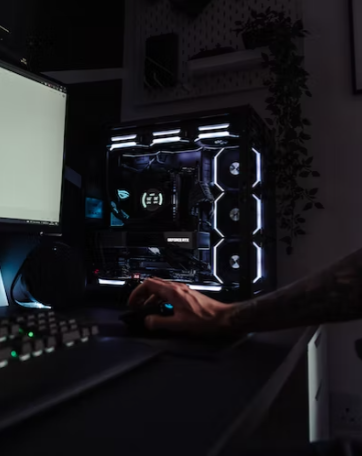## Custom PC

During an intense video game session, a custom computer produces 1,000 W of heat. If it is drawing 5 A of current, what is the total resistance (Ω) of all its internal components?Expand Hint
Power absorbed by a resistive element:
$$P=VI=\frac{V^{2}}{R}=I^2R$$$where $$V$$ is voltage, $$I$$ is current, and $$R$$ is resistance. Hint 2 Based on the problem statement’s givens, solve for current using the equation: $$P=I^2R$$$
Power absorbed by a resistive element:
$$P=VI=\frac{V^{2}}{R}=I^2R$$$where $$V$$ is voltage, $$I$$ is current, and $$R$$ is resistance. Solving for Resistance using the problem statement’s knowns: $$P=I^2R\to 1,000W=(5A)^2\times R$$$
$$R= \frac{1,000W}{25A}=40\:\Omega$$\$
40 Ω
Similar Problems from FE Sub Section: Power Absorbed by a Resistive Element

Similar Problems from FE Section: Electrostatics